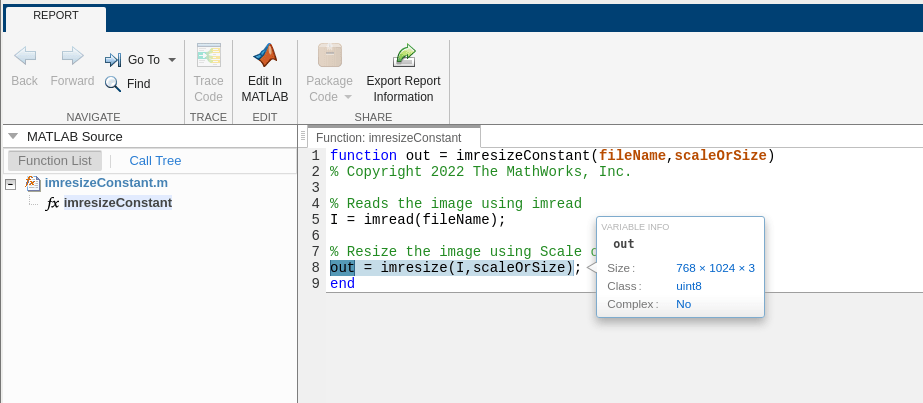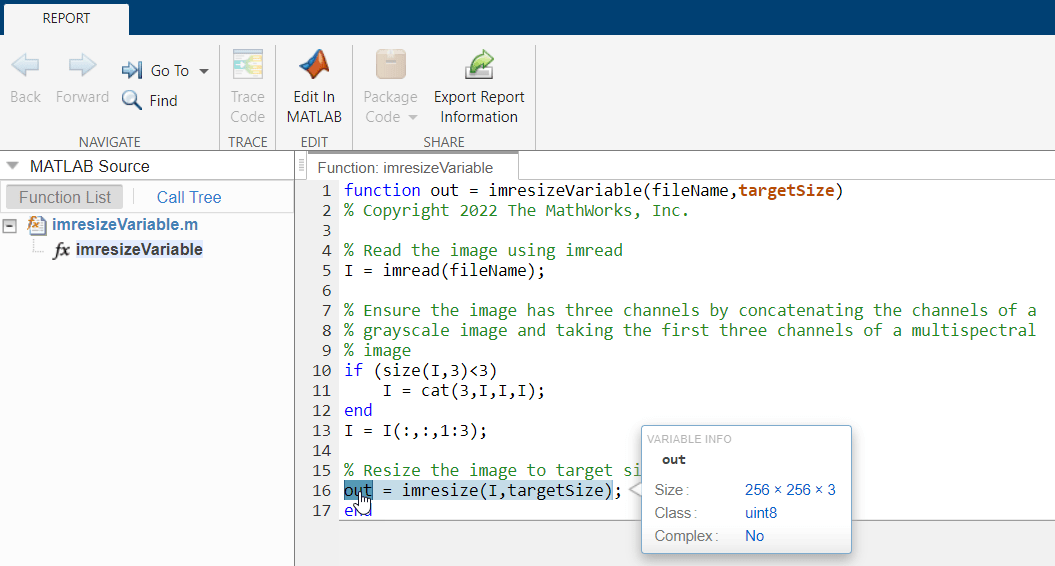# Generate Code to Resize Images to Fixed Output Size

This example shows how to generate a MEX file containing C code that resizes images to a consistent output size.

The `imresize` function offers two options for resizing 2-D images: rescaling by a scale factor, and resizing to a target output size. To convert a MATLAB function to C or C++ code, you can use the `codegen` function. This example shows how to generate a MEX file that resizes images in three scenarios:

1. Resize a specific image file with a filename that does not vary.

2. Resize any image file. When resizing different image files, the filenames can vary and the images can have different input sizes.

3. Resize a batch of specified input image files, which can vary and have different input sizes.

In all three scenarios, the example uses a constant scale factor or target size to ensure that the output size is consistent.

### Scenario 1: Generate Code to Resize Constant Input Image

In this scenario, you want to generate code that resizes a single image with a fixed filename. The input image is a constant input to the `codegen` function because the value of the argument is always the same. Specify the filename of the image.

`imageFileName = "foggyroad.jpg";`

#### Define Entry-Point Function

The MATLAB function that you want to convert is called the entry-point function. In this scenario, the entry-point function is a custom function called `imresizeConstant`. The `imresizeConstant` function reads a single image from a file and then resizes the image using the `imresize` function. The function supports both rescaling by an isotropic scale factor and resizing both image dimensions to a target size.

• To resize an image by an isotropic scale factor, specify the `scaleOrSize` input argument as a scalar.

• To resize both dimensions to a target size, specify the `scaleOrSize` input argument as a two-element vector of the form [numrows numcols].

`type imresizeConstant.m`
```function out = imresizeConstant(fileName,scaleOrSize) % Copyright 2022 The MathWorks, Inc. % Reads the image using imread I = imread(fileName); % Resize the image using Scale or Size out = imresize(I,scaleOrSize); end ```

#### Option 1: Resize Using Constant Scale Factor

Specify the scale factor.

`scaleFactor = 0.5;`

Generate the MEX file using the `codegen` function. You must define the data type of each input argument of the entry-point function at compile time because the C and C++ coding languages use static typing. For this scenario, you can further specify both input arguments as constant values using the `coder.Constant` class. For more information, see Specify Properties of Entry-Point Function Inputs (MATLAB Coder).

`codegen -config:mex imresizeConstant.m -args {coder.Constant(imageFileName),coder.Constant(scaleFactor)} -report`
```Code generation successful: To view the report, open('codegen/mex/imresizeConstant/html/report.mldatx') ```

To open the code generation report, click `View report`. In the `Function` pane, the orange font color of both input arguments indicates that they are constant arguments to the entry-point function. If you pause on the `out` variable, the Variable Info tooltip indicates that `out` has a fixed size, as expected. The size is equal to one half of the input size. Because the input image does not change, the output image size is consistent each time you run the MEX file.#### Option 2: Resize Using Constant Target Size

Specify a constant target size.

`targetSize = [256 256];`

Generate the MEX file using the `codegen` function. Define the input argument types, and use the `coder.Constant` class to specify both inputs as constant values. The compiled function resizes the input image to a target size because `targetSize` is a vector.

`codegen -config:mex imresizeConstant.m -args {coder.Constant(imageFileName),coder.Constant(targetSize)} -report`
```Code generation successful: To view the report, open('codegen/mex/imresizeConstant/html/report.mldatx') ```

Open the code generation report. In the `Function` pane, the orange font color indicates that both inputs are constant. If you pause on the `out` variable, the Variable Info tooltip indicates that `out` has a fixed size, equal to the target size specified by `targetSize`.### Scenario 2: Generate Code to Resize Variable Input Image

In this scenario, you want to generate code that accepts any input image filename for resizing. The input image is a variable input to the `codegen` function because the value of the argument can change.

To ensure that different input images always have the same output size, you must resize using a constant target size. Rescaling by a scale factor does not guarantee that the output images will have a fixed size, because input images with different sizes will yield different output sizes.

#### Define Entry-Point Function

For this scenario, define a new entry-point function `imresizeVariable.m`. This function ensures that the resized image has three channels. If the input image is a grayscale image, the function concatenates three copies of the image along the third dimension. If the input image is a multispectral image or volume, the function preserves only the first three channels.

`type imresizeVariable.m`
```function out = imresizeVariable(fileName,targetSize) % Copyright 2022 The MathWorks, Inc. % Read the image using imread I = imread(fileName); % Ensure the image has three channels by concatenating the channels of a % grayscale image and taking the first three channels of a multispectral % image if (size(I,3)<3) I = cat(3,I,I,I); end I = I(:,:,1:3); % Resize the image to target size out = imresize(I,targetSize); end ```

#### Resize Using Constant Target Size

Specify a constant target size to use as input to the entry-point function.

`targetSize = [256 256];`

Generate the MEX file using the `codegen` function. Specify a variable filename of data type `string`, and a `coder.Constant` class for the target output size.

`codegen -config:mex imresizeVariable.m -args {imageFileName,coder.Constant(targetSize)} -report`
```Code generation successful: To view the report, open('codegen/mex/imresizeVariable/html/report.mldatx') ```

Open the code generation report. In the `Function` pane, the orange font color indicates that only `targetSize` is constant, while `fileName` can vary. If you pause on the `out` variable, the Variable Info tooltip indicates that all three dimensions of the output image are fixed, as desired.Note that if you accidentally specify a scale factor instead of a target size, the Variable Info tooltip displays a question mark for one or more of the dimensions. The question mark indicates that the size of the output image is not fixed.### Scenario 3: Generate Code to Resize Multiple Input Images

In this scenario, you want to generate code that accepts multiple input image filenames for resizing. The input image is a variable input to the `codegen` function because the value of the argument can change.

To ensure that different input images always have the same output size, you must resize using a constant target size. Specify the filenames of the image.

```imageFileName1 = "foggyroad.jpg"; imageFileName2 = "foosball.jpg"; imFileNames = {imageFileName1,imageFileName2};```

#### Define Entry-Point Function

Define an entry-point function named `imresizeVariableBatch.m` that reads a batch of input images specified as a cell array, and resizes each image to a target size. The function returns the resized images as a 4-D array, where the fourth dimension corresponds to the number of input images.

`type imresizeVariableBatch.m`
```function out = imresizeVariableBatch(imageFileNameCellArray,targetSize) % Copyright 2022 The MathWorks, Inc. numImages = coder.const(numel(imageFileNameCellArray)); % Allocate memory for output image out = coder.nullcopy(zeros([targetSize,3,numImages],"uint8")); % For each image in the input cell array, read and resize the image. for i = coder.unroll(1:numImages) I = imread(imageFileNameCellArray{i}); if (size(I,3)<3) I = cat(3,I,I,I); end I = I(:,:,1:3); out(:,:,:,i) = imresize(I,targetSize); end end ```

#### Resize Using Constant Target Size

Specify a constant target size to use as input to the entry-point function.

`targetSize = [256 256];`

Generate the MEX file using the `codegen` function. Specify the first input argument as a cell array containing the filenames. The `codegen` function configures the MEX file to accept a cell array of two strings, which can vary. Specify a `coder.Constant` class for the target output size.

`codegen -config:mex imresizeVariableBatch.m -args {imFileNames,coder.Constant(targetSize)} -report`
```Code generation successful: To view the report, open('codegen/mex/imresizeVariableBatch/html/report.mldatx') ```

Open the code generation report. In the `Function` pane, the orange font color indicates that only `targetSize` is constant, while `imageFileNameCellArray` can vary. If you pause on the `out` variable, the Variable Info tooltip indicates that all dimensions of the 4-D output image array are fixed.